Technical Article

# How to Use Scilab to Analyze Frequency-Modulated RF Signals

September 11, 2018 by Robert Keim

## Computing a discrete Fourier transform can help you to analyze the ways in which RF modulation affects the spectrum of a carrier signal.

Computing a discrete Fourier transform can help you to analyze the ways in which RF modulation affects the spectrum of a carrier signal.

### Previous Articles on Scilab-Based Digital Signal Processing

The frequency-domain effects of amplitude modulation are fairly straightforward: the fundamental mathematical operation in an AM system is multiplication, and multiplication causes a spectrum to shift such that it is centered on a new frequency. The mathematical relationship that forms the basis of frequency modulation is more complicated:

$$x_{FM}(t)=\sin(\omega_Ct+\int_{-\infty}^{t} x_{BB}(t)dt)$$

As you can see, a frequency-modulated signal is created by adding the integral of the baseband signal to the argument of the sine function that corresponds to the carrier. In other words, the carrier is sin(ωCt), meaning that it is a sine wave with angular frequency ωC and no phase term, and the FM waveform is the carrier with the addition of a time-varying phase term equal to the integrated baseband signal.

Phase modulation is closely related to frequency modulation:

$$x_{PM}(t)=\sin(\omega_Ct+x_{BB}(t))$$

Thus, if you want to analyze a phase-modulated signal, almost everything in this article will be applicable. All you have to do is use the baseband signal, instead of the integral of the baseband signal, as the time-varying phase term.

### Integrating the Baseband Signal

Let’s start by creating the baseband and carrier arrays. Note that the sampling frequency and the buffer length have increased by a factor of ten compared to what we used in the previous article; I did this because I wanted the higher-frequency portions of the modulated waveform to have more samples per cycle.

BasebandFrequency = 10e3;
CarrierFrequency = 100e3;
SamplingFrequency = 1e7;
BufferLength = 2000;
n = 0:(BufferLength - 1);
BasebandSignal = sin(2*%pi*n / (SamplingFrequency/BasebandFrequency));
CarrierSignal = sin(2*%pi*n / (SamplingFrequency/CarrierFrequency));
plot(n, BasebandSignal)
plot(n, CarrierSignal)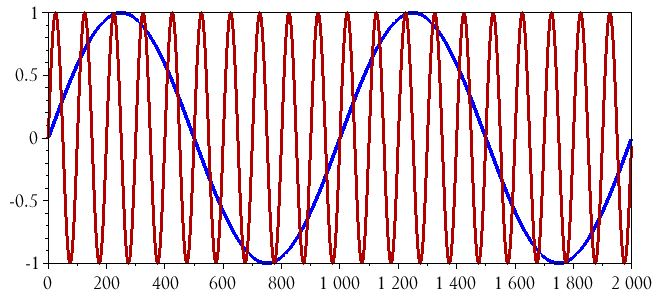Now we need to integrate the baseband signal. Computing an indefinite integral of a digitized waveform is not particularly straightforward. Scilab does have a command, called integrate(), that can help us with that task, but integrate() is almost a topic unto itself, and consequently I’m going to use a simpler method in this article and discuss the use of the integrate() command in the next article.

The simpler method that we’ll use for the time being is based on the following observations:

1. The baseband signal is a uniform, single-frequency sine wave.
2. The indefinite integral of a sine wave is a negative cosine wave (plus a constant; in our case the constant will be zero).

So all we have to do is change the BasebandSignal = sin(...) command to BasebandSignal_integral = –cos(...):

BasebandSignal_integral = -cos(2*%pi*n / (SamplingFrequency/BasebandFrequency));
plot(n, BasebandSignal)
plot(n, BasebandSignal_integral)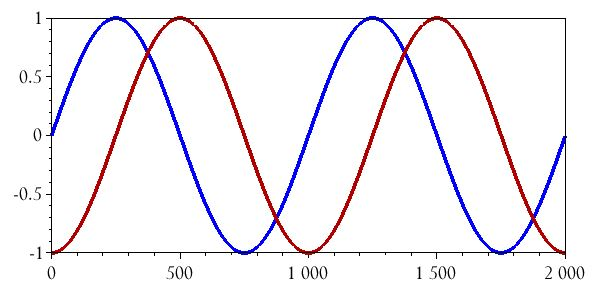### Frequency Modulation in the Time Domain

Now we are ready to generate the FM signal. All we have to do is take the command used to create the carrier waveform and add the array BasebandSignal_integral to the argument of the sin() function.

ModulatedSignal_FM = sin((2*%pi*n / (SamplingFrequency/CarrierFrequency)) + BasebandSignal_integral);

Here is the result:

plot(n, ModulatedSignal_FM)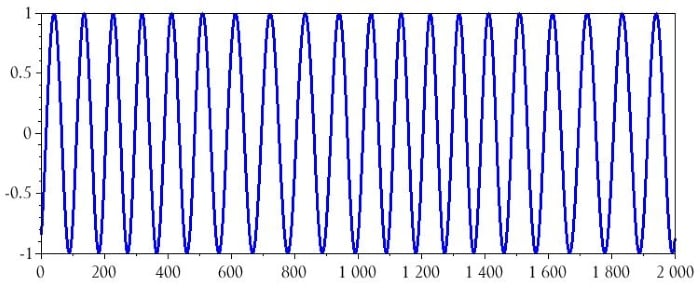Don’t worry, the frequency modulation is in there somewhere. The problem is, you can’t see it because the frequency variations are too small relative to the carrier frequency. This is where the modulation index comes in. The modulation index, denoted by m, is used to increase (or decrease) the amount of frequency variation caused by a given baseband value:

$$x_{FM}(t)=\sin(\omega_Ct+m\int_{-\infty}^{t} x_{BB}(t)dt)$$

If we incorporate a modulation index of 4 into the command used to generate the FM data, the effect of the modulation is much more apparent:

ModulatedSignal_FM = sin((2*%pi*n / (SamplingFrequency/CarrierFrequency)) + (4*BasebandSignal_integral));
plot(n, ModulatedSignal_FM)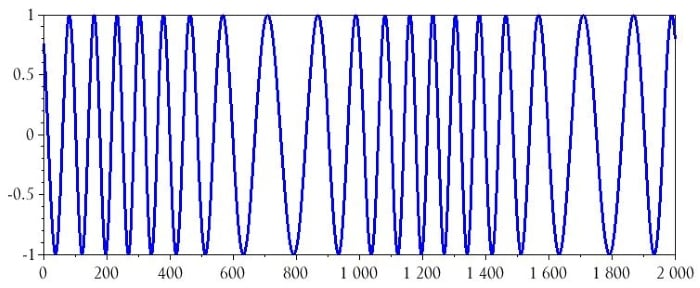We can add the baseband and the integrated baseband into the plot, just in case you want to ponder the relationship between these two signals and the FM waveform.

plot(n, BasebandSignal)
plot(n, BasebandSignal_integral)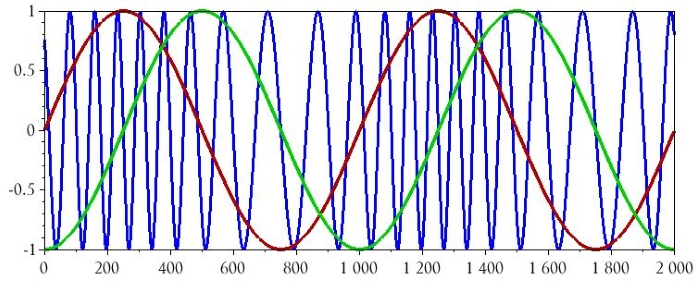### FM in the Frequency Domain

The following commands will produce a frequency-domain representation of the FM signal.

HalfBufferLength = BufferLength/2;
HorizAxisIncrement = (SamplingFrequency/2)/HalfBufferLength;
DFTHorizAxis = 0:HorizAxisIncrement:((SamplingFrequency/2)-HorizAxisIncrement);
FM_DFT = fft(ModulatedSignal_FM);
FM_DFT_magnitude = abs(FM_DFT);
plot(DFTHorizAxis, FM_DFT_magnitude(1:HalfBufferLength))
xlabel("Frequency (Hz)")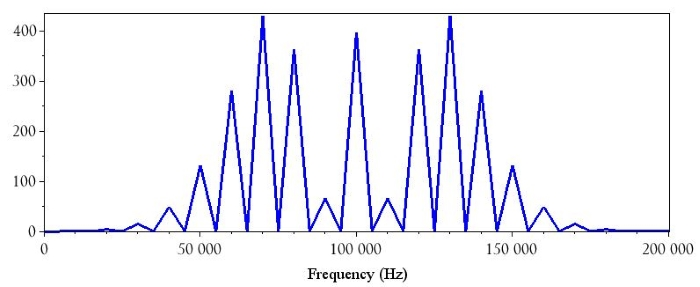There are two characteristics here that I want to mention: First, the sideband amplitude can be higher than the amplitude of the component at the carrier frequency. Second, the modulated bandwidth (about ±70 kHz relative to the carrier frequency) is much larger than the bandwidth of the baseband signal (i.e., ±10 kHz).

It’s important to understand, however, that the specific features shown above are not present in all cases of frequency modulation. Various factors affect the characteristics of FM spectra; for example, if we lower the modulation index to 2, we get the following: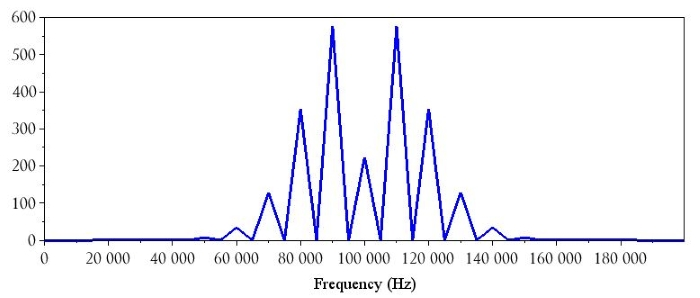If we return the modulation index to 4 and then reduce the baseband frequency by a factor of 2, the spectrum changes to this: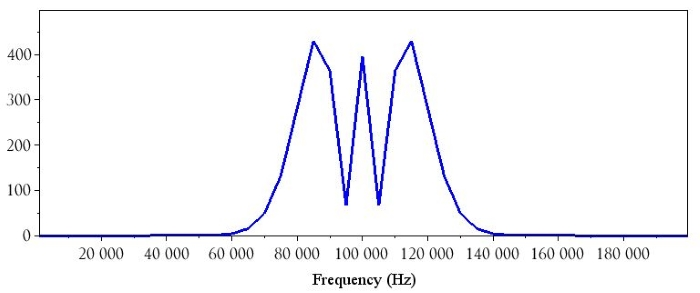### Conclusion

I haven’t extensively studied frequency modulation from the perspective of theoretical analysis, but as far as I can tell it is quite difficult to predict the characteristics of an FM spectrum based on mathematical relationships between the baseband and the carrier. This is a great reason to use Scilab (or MATLAB, or Octave) for frequency-domain analysis of FM systems. I hope that this article has provided a good introduction, and we’ll continue the discussion in the next article.